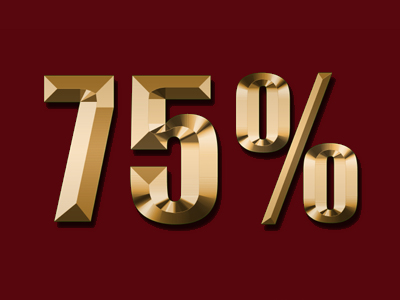75% is exactly the same as 34

# Percentages 2 (Difficult)

Welcome to the second of our difficult Eleven Plus maths quizzes on percentages. In the first, you were asked to express one number as a percentage (or proportion) of another – I hope you didn’t find it too hard! In this quiz, we’ll offer you more chance to practice.

There is a simple way to do it. Here’s an example:

What percentage of 20 is 4?
First, arrange the problem like this :
(4 ÷ 20) × 100%
Next, you can make it easier by turning it around, like this:
(4 × 100) ÷ 20
Work out the part in brackets:
400 ÷ 20
This gives you the answer = 20%

Just follow this formula and percentages should be simple. So, now you are ready, let’s begin. Good luck!

1.
Of the 12,500 spectators at a football match, 1,000 came to support the away team and the rest came to support the home team. What percentage of the crowd followed the home team?
92%
64%
36%
8%
(1,000 ÷ 12,500) x 100% = (1,000 × 100) ÷ 12,500 = 8%.
This tells you the proportion of away fans. To find the proportion of home fans, simply subtract 8 from 100:
100 – 8 = 92%
2.
If 7 pupils in a class of 28 fail their maths test, what percentage of the class does this represent?
15%
20%
25%
33.33%
(7 ÷ 28) x 100% = (7 × 100) ÷ 28 = 25%
3.
What percentage of 16 is 72?
22.22%
450%
44.44%
400%
(72 ÷ 16) x 100% = (72 × 100) ÷ 16 = 450%.
In ‘top-heavy’ fractions (e.g. 7216), the percentage will be more than 100%
4.
A company has a workforce of 748. Of these, 68 work in the offices and the rest work on the shop floor. What percentage of the workforce work in the offices?
15.15%
12.12%
9.09%
6.06%
(68 ÷ 748) x 100% = (68 × 100) ÷ 748 = 9.09%
5.
What percentage of 300 is 15?
3%
5%
7%
9%
(15 ÷ 300) × 100% = (15 × 100) ÷ 300 = 5%
6.
If, in a survey on holiday destinations, 24 out of 1,000 people said they went to Canada for their holiday this year, what percentage does this represent?
24%
2.4%
0.24%
0.024%
To convert parts of 1,000 to percentages, simply divide by 10
7.
What percentage of 250 is 187.5?
85%
80%
75%
70%
(187.5 ÷ 250) x 100% = (187.5 × 100) ÷ 250 = 75%.
Decimal numbers are surprisingly easy to work with – multiplying them by 100 just means moving the digits two places to the left
8.
What percentage of 56 is 12?
30%
27.33%
25%
21.43%
(12 ÷ 56) x 100% = (12 × 100) ÷ 56 = 21.43%
9.
What percentage of 270 is 54?
27.5%
25%
22.5%
20%
(54 ÷ 270) x 100% = (54 × 100) ÷ 270 = 20%
10.
If 2 out of 12 people have green eyes, what percentage does this represent?